# Biotechnology Engineering - BT 2013 GATE Paper (Practice Test)

## 65 Questions MCQ Test GATE Past Year Papers for Practice (All Branches) | Biotechnology Engineering - BT 2013 GATE Paper (Practice Test)

Description
Attempt Biotechnology Engineering - BT 2013 GATE Paper (Practice Test) | 65 questions in 180 minutes | Mock test for GATE preparation | Free important questions MCQ to study GATE Past Year Papers for Practice (All Branches) for GATE Exam | Download free PDF with solutions
QUESTION: 1

Solution:
QUESTION: 2

Solution:
QUESTION: 3

### Mahatama Gandhi was known for his humility as

Solution:
QUESTION: 4

"All engineering students" "should learn mechanics", "mathematics and" "how to do computation."
I                                          II                                 III                                 IV
Which of the above underlined parts of the sentence is not appropriate?

Solution:
QUESTION: 5

Select the pair that best expresses a relationship similar to that expressed in the pair:
water: pipe::

Solution:
QUESTION: 6

Q. 6 to Q.10 carry two marks each.

Velocity of an object fired directly in upward direction is given by V=80-32t, where t (time) is in seconds. When will the velocity be between 32 m/sec and 64 m/sec?

Solution:
QUESTION: 7

In a factory, two machines M1 and M2 manufacture 60% and 40% of the autocomponents respectively. Out of the total production, 2% of M1 and 3% of M2 are found to be defective. If a randomly drawn autocomponent from the combined lot is found defective, what is the probability that it was manufactured by M2?

Solution:
QUESTION: 8

Following table gives data on tourists from different countries visiting India in the year 2011.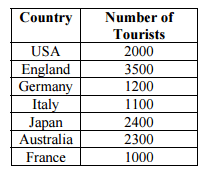Which two countries contributed to the one third of the total number of tourists who visited India in 2011?

Solution:
QUESTION: 9

If |-2x+9| =3 then the possible value of |-x| -x2 would be:

Solution:
QUESTION: 10

All professors are researchers
Some scientists are professors

Which of the given conclusions is logically valid and is inferred from the above arguments:

Solution:
QUESTION: 11

Q. 11 – Q. 35 carry one mark each.
Under alkaline conditions, DNA is more stable than RNA because

Solution:
QUESTION: 12

Which one of the following modifications is common toboth protein and DNA?

Solution:
QUESTION: 13

Protein A, which has strong affinity to Fc region of immunoglobulin, is extracted from

Solution:
QUESTION: 14

The first humanized monoclonal antibody approved for the treatment of breast canceris

Solution:
QUESTION: 15

Which one of the following aminoacids in proteins does NOT undergo phosphorylation?

Solution:
QUESTION: 16

The role of an adjuvant isto

Solution:
QUESTION: 17

Endogenous antigens are presented on to the cell surface along with

Solution:
QUESTION: 18

Human genome sequencing project involved the construction of genomic library in

Solution:
QUESTION: 19

The nucleotide analogue used in DNA sequencing by chain termination method is

Solution:
QUESTION: 20

In nature, the horizontal gene transfer across bacteria is mediated by

Solution:
QUESTION: 21

Phylum proteobacteria is subdivided into α-, β-, γ-, δ- and ε-proteobacteria based on

Solution:
QUESTION: 22

Which one of the following is an ABC transporter?

Solution:
QUESTION: 23

The catalytic efficiency for an enzyme is definedas

Solution:
QUESTION: 24

Of the two diploid species, species I has 36 chromosomes and species II has 28 chromosomes. How many chromosomes would be found in an allotriploid individual?

Solution:
QUESTION: 25

The RNA primer synthesized during the replication process in bacteria is removed by

Solution:
QUESTION: 26

The suitable substitution matrix to align closely related sequences is

Solution:
QUESTION: 27

If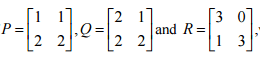which one of the following statements is TRUE?

Solution:
QUESTION: 28

If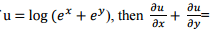Solution:
QUESTION: 29

Hypophosphatemia is manifested by an X-linked dominant allele.What proportion of the offsprings from a normal male and an affected heterozygous female will manifest the disease?

Solution:
QUESTION: 30

One of theeigen values of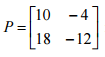Solution:
*Answer can only contain numeric values
QUESTION: 31

Q. 31 – Q. 35 are of numerical answer type.

A callus of 5 g dry weight was inoculated on semi-solid medium for growth. The dry weight of the callus was found to increase by 1.5 fold after 10 days of inoculation. The growth index of the culture is _________

Solution:
*Answer can only contain numeric values
QUESTION: 32

A chemostat is operated at a dilution rate of 0.6 h-1. At steady state, the biomass concentration in the exit stream was found to be 30 g l-1. The biomass productivity (g l-1h-1) after 3h of steady state operation will be ___________

Solution:
*Answer can only contain numeric values
QUESTION: 33

A batch bioreactor is to be scaled up from 10 to 10,000 liters. The diameter of the large bioreactor is 10 times that of the small bioreactor. The agitator speed in the small bioreactor is 450 rpm. Determine the agitator speed (rpm) of the large bioreactor with same impeller tip speed as that of the small bioreactor. _________

Solution:
*Answer can only contain numeric values
QUESTION: 34

Calculate the percentage sequence identity for the pairwise alignment given below. _______

H E L L O – Y E L L O W

Solution:
*Answer can only contain numeric values
QUESTION: 35

In a batch culture, the specific rate of substrate utilization is 0.25 g (g cell mass)-1 h-1 and specific rate of product formation is 0.215 g (g cell mass)-1 h-1. Calculate the yield of product from the substrate(Yp/s). ___________

Solution:
QUESTION: 36

Q. 36 to Q. 65 carry two marks each.

Match the commercial microbial sources in Group I with the products in Group II.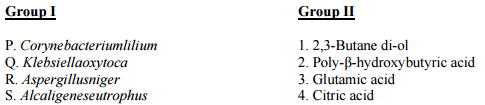Solution:
QUESTION: 37

Match the entries in the Group I with the elution conditions in Group II.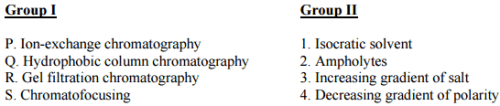Solution:
QUESTION: 38

Determine the correctness or otherwise of the following Assertion (a) and Reason (r).

Assertion: Immobilization of plant cells can enhance secondary metabolite production during bioreactor cultivation.

Reason: Immobilization protects the plant cells from shear forces in the bioreactor.

Solution:
QUESTION: 39

Match the cell structures in Group I with the organismsin Group II.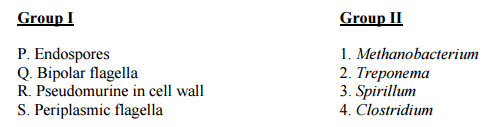Solution:
QUESTION: 40

Match the antibioticsinGroup Iwith the targets inGroup II.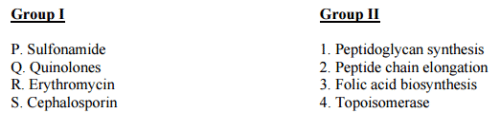Solution:
QUESTION: 41

In nature, Agrobacterium tumefaciens mediated infection of plant cells leads to

P. crown gall disease in plants

Q. hairy root disease in plants

R. transfer of T-DNA into the plant chromosome

S. transfer of Ri-plasmid into the plant cell

Solution:
QUESTION: 42

Match the entries inGroup I with the enzymes inGroup II.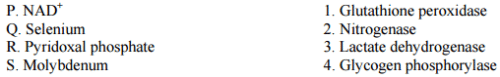Solution:
QUESTION: 43

Match the herbicides in Group Iwith the target enzymesin Group II.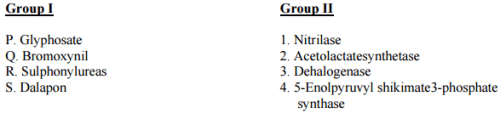Solution:
QUESTION: 44

The activity of an enzyme was measured by varying the concentration of the substrate (S) in the presence of three different concentrations of inhibitor (I) 0, 2 and 4 mM.The double reciprocal plot given belowsuggests that the inhibitor (I) exhibits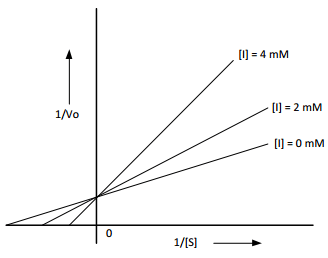Solution:
QUESTION: 45

Match the entries in Group Iwith the entries in Group II.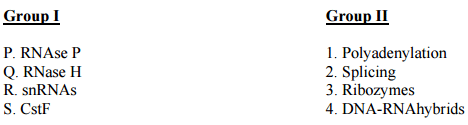Solution:
QUESTION: 46

Determine the correctness or otherwise of the following Assertion (a) and Reason (r).

Assertion:UPGMA method produces ultrametric tree.

Reason:Sequence alignment is converted into evolutionary distances in UPGMA method.

Solution:
QUESTION: 47

Match the entries in the Group Iwith the entries inGroup II.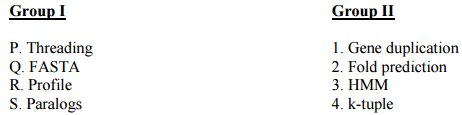Solution:
QUESTION: 48

Evaluate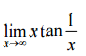Solution:
QUESTION: 49

The Laplace transform of f(t)=2t+6 is

Solution:
QUESTION: 50

The solution of the following set of equations is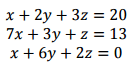Solution:
QUESTION: 51

The solution to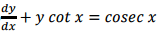is

Solution:
QUESTION: 52

A complete restriction digestion of a circular plasmid (5000bp) was carried out with HindIII,BamHIandEcoRIindividually. Restriction digestion yielded following fragments.

Plasmid + HindIII→ 1200bp and3800bp

Plasmid + BamHI→5000bp

Plasmid + EcoRI→ 2500bp

The number of sites for EcoRI,BamHIandHindIIIpresent on this plasmid are

Solution:
*Answer can only contain numeric values
QUESTION: 53

Q. 53 – Q. 57 are of numerical answer type.

The total number of fragments generated by the complete and sequential cleavage of the polypeptide given below by Trypsin followed by CNBr is _________

Phe-Trp-Met-Gly-Ala-Lys-Leu-Pro-Met-Asp-Gly-Arg-Cys-Ala-Gln

Solution:
*Answer can only contain numeric values
QUESTION: 54

In a genetic study, 80 people were found to have alleles for polydactyly. Only 36 of them were polydactylous. What is the extent of penetrance percentage? ________

Solution:
*Answer can only contain numeric values
QUESTION: 55

One percent of the cars manufactured by a company are defective. What is the probability (upto four decimals) that more than two cars are defective, if 100 cars are produced? __________

Solution:
*Answer can only contain numeric values
QUESTION: 56

The maximum cell concentration (g l-1) expected in a bioreactor with initial cell concentration of 1.75 g l-1 and an initial glucose concentration of 125 g l-1 is (Yx/s = 0.6 g cell/g substrate) ________

Solution:
*Answer can only contain numeric values
QUESTION: 57

A fed batch culture was operated with intermittent addition of glucose solution at a flow rate of 200 ml h-1. The values of Ks, um and D are 0.3 g l-1, 0.4 h-1 and 0.1 h-1, respectively. Determine the concentration of growth limiting substrate (gl-1) in the reactor at quasi-steady state. ____________

Solution:
QUESTION: 58

Common Data Questions

Common Data for Questions 58 and 59:

A solution was prepared by dissolving 100 mg of protein X in 100 ml of water. Molecular weight of protein X is 15,000 Da; Avogadro’s number = 6.022x 1023.

Calculate the molarity(μM) of the resulting solution.

Solution:
QUESTION: 59

A solution was prepared by dissolving 100 mg of protein X in 100 ml of water. Molecular weight of protein X is 15,000 Da; Avogadro’s number = 6.022x 1023.

The number of moleculespresent in this solution is

Solution:
QUESTION: 60

Common Data for Questions 60 and 61:

The binding efficiency of three different receptorsR1, R2 and R3 were tested against a ligand using equilibrium dialysis, with a constant concentration of receptor and varying concentrations of ligand. The Scatchard plot of receptor titration with different concentration of ligand is given below (ris moles of bound ligand per moles of receptor and c is concentration of free ligand)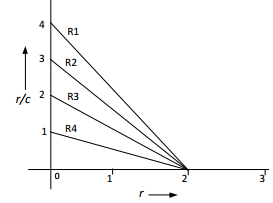The number of ligand binding sites present on receptors R1 and R3, respectively are

Solution:
QUESTION: 61

The binding efficiency of three different receptorsR1, R2 and R3 were tested against a ligand using equilibrium dialysis, with a constant concentration of receptor and varying concentrations of ligand. The Scatchard plot of receptor titration with different concentration of ligand is given below (ris moles of bound ligand per moles of receptor and c is concentration of free ligand)Which one of the receptors has thehighestaffinity for the ligand?

Solution:
QUESTION: 62

A DNA fragment of 5000bp needs to be isolated from E.coli (genome size 4x103 kb) genomic library.

The minimum number of independent recombinant clones required to represent this fragment in genomic library are

Solution:
QUESTION: 63

A DNA fragment of 5000bp needs to be isolated from E.coli (genome size 4x103 kb) genomic library.

The number of clones to represent this fragment in genomic library with a probability of 95% are

Solution:
QUESTION: 64

During sterilization of a fermentation medium in a given bioreactor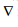heating= 12.56,cooling = 7.48 and the total value ofrequired for whole sterilization process is 52, whereis the design criteria.

What is the value ofholding?

Solution:
QUESTION: 65

During sterilization of a fermentation medium in a given bioreactorheating= 12.56,cooling = 7.48 and the total value ofrequired for whole sterilization process is 52, whereis the design criteria.

What is the holding period (min) at a k value of 3.36min-1?

Solution:Use Code STAYHOME200 and get INR 200 additional OFF Use Coupon Code